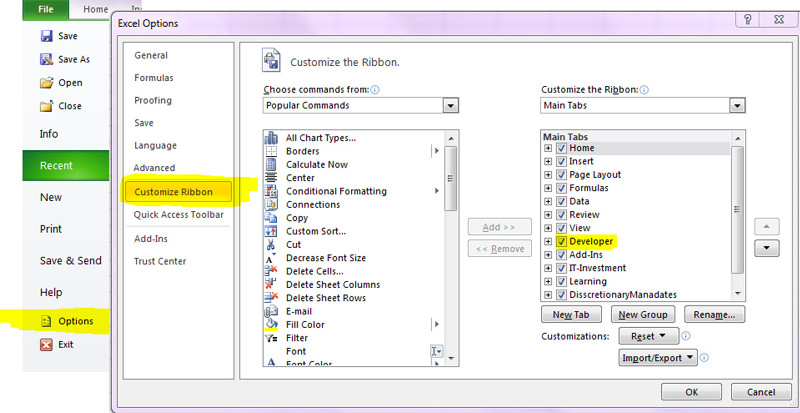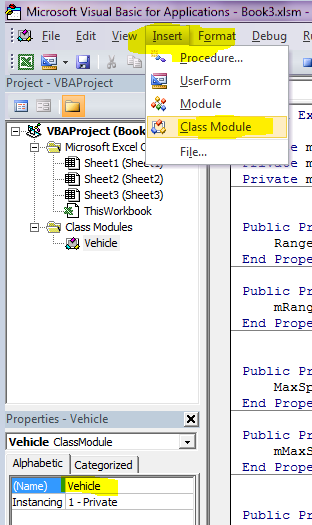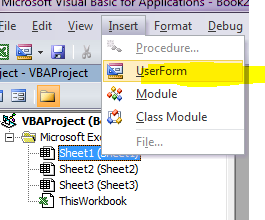VBA

Some notes about Visual Basic for Applications (VBA) in Excel

First steps

Enable Developer tools TabStart Macro Recorder Developer - Record Macro During the recording you can switch between absolute and relative cell address recording (cell A2 vs offset)

Start a macro Define a shortcut for the macro Developer - Insert - Button, assign Macro

Object browser Developer - Visual Basic - View - Object Browser

Immediate window

?1+1
2

Code

Data types

Dim bytAge as Byte

Option Explicit

Static

If a method contains a static variable it will remmeber its value for the next time the method is called

Static intCountMe As Integer

Public

If variables are declared with Public instead of Dim they can be accessed globally Public intAge as Integer

Const

Variables that cannot be changed after they have be initialized. There are already a lot of constants defined, which usually start with vb or xl.

Val("42")
Str(42)
IsNumeric("42")

Set foo= Nothing

Arrays

Dim arrMyArray(0 To 5) As String

arrMyArray(0) = "Zero"
arrMyArray(1) = "One"
arrMyArray(2) = "Two"

Dim s As Variant

For Each s In arrMyArray
Debug.Print s
Next s

More than one array dimension

Dim arrTwoDimensions(0 To 15, 0 To 15) As Boolean
arrTwoDimensions(0, 0) = False

Change Array size

Dim arrMyArray(1) As String
arrMyArray(0) = "Zero"

ReDim arrMyArray(2)
arrMyArray(0) = "Zero"
arrMyArray(1) = "One"

ReDim Preserve arrMyArray(5)
Debug.Print LBound(arrMyArray)
Debug.Print UBound(arrMyArray)

Show debug messages

Debug.Print myVariable

if statement

If intMyNumber = 1 Then
Debug.Print "1"
ElseIf intMyNumber = 2 Then
Debug.Print "2"
Else
Debug.Print "?"
End If

Case Statement

Select Case intMyNumber
Case 1
Debug.Print "1"
Case 2
Debug.Print "2"
Case 3 To 8
Debug.Print "3..8"
Case Else
Debug.Print "?"
End Select

Loops

For loop

For i = 10 To 1 Step -1
Debug.Print i
If i = 5 Then
Exit For
End If
Next i

While loop

Do While i < 100
i = i + 1
Debug.Print i
Loop
While i < 100
i = i + 1
Debug.Print i
Wend

With

If want to access more than one attribute of an object you can use the with statement

With FOO
.age = 3
.enable = True
.Name = "Foo"
End With

Methods

Sub Foo(ByVal a As Integer, ByRef b As Integer)
a = -1 ' ByVal will not have an effect outside this method
b = -2 ' ByRef will have an effect outside this method
End Sub

Functions

Function fact(start As Integer) As Integer
If start > 0 Then
fact = start * fact(start - 1)
Else
fact = 1
End If
End Function

Debug.Print fact(5) ‘ 120

Functions can not only be used in VBA code but also directly in Excel cells. So you could also insert in an Excel cell

=FACT(5)

Non fixed number of parameters

Sub Sort(inverse As Boolean, ParamArray data() As Variant)

Classes

You can add new classes view VBA - Insert - Class ModuleIn the Properties field, the name of the class can be defined.If you want to add Functions or Property methods (getters and setters) use Insert - Add Procedure

Example for a simple class

Option Explicit

Private mName As String
Private mRange As Long
Private mMaxSpeed As Integer

' Initialize object
Private Sub Class_Initialize()
mName = "Unnamed"
mRange = 0
mMaxSpeed = 0
End Sub

' Setter and Getter Range
Public Property Get Range() As Variant
Range = mRange
End Property
Public Property Let Range(ByVal vNewValue As Variant)
mRange = vNewValue
End Property

' Setter and Getter MaxSpeed
Public Property Get MaxSpeed() As Variant
MaxSpeed = mMaxSpeed
End Property

Public Property Let MaxSpeed(ByVal vNewValue As Variant)
mMaxSpeed = vNewValue
End Property

' Setter and Getter VehicleName
Public Property Get VehicleName() As Variant
Name = mName
End Property

Public Property Let VehicleName(ByVal vNewValue As Variant)
mName = vNewValue
End Property

' Output method
Public Sub output()
Debug.Print mName
Debug.Print mRange
Debug.Print mMaxSpeed
End Sub

Example how to use this class

Dim car As New Vehicle
car.output
car.VehicleName = "Volkswagen"
car.MaxSpeed = 180
car.Range = 650
car.output
Set car = Nothing

Date and time

Dim t As Date
t = TimeSerial(Second:=59, Minute:=59, Hour:=23)
Debug.Print t
Debug.Print Hour(t)
Debug.Print Minute(t)
Debug.Print Second(t)

Dim d As Date
d = DateSerial(Day:=31, Month:=12, Year:=2013)
Debug.Print d
Debug.Print Day(d)
Debug.Print Month(d)
Debug.Print Year(d)

Random number

Rnd gives you a random number between 0 and 1, but may be used to get you random int numbers as well

Function Random(min As Integer, max As Integer) As Single
Application.Volatile
If (min <= max) Then
Random = Int((Rnd * (max - min + 1)) + min)
End If
End Function

Error Handling

You can ignore any error (not recommended), jump with goto if an error occurs or raise your own errors

Sub MySub()
Dim intMyNumber As Integer

' ignore possible errors
On Error Resume Next
' Error: String vs Integer
intMyNumber = "abc"
' enable error tracking again
On Error GoTo 0

On Error GoTo MyError
intMyNumber = "a42"
On Error GoTo 0

Debug.Print "This is the value of intMyNumber"
Debug.Print intMyNumber

Err.Raise 1003

Exit Sub

' Jump here if you got an error

MyError:
Debug.Print "MyError was called after an error occured: ",
Debug.Print Err.Number,
Debug.Print " ",
Debug.Print Err.Description,
Debug.Print " ",
Debug.Print Err.Source
intMyNumber = 42
Resume Next
End Sub

Excel VBA

Iterate through Excel cells

Dim rngMyRange As Range
Dim r As Range
Dim ws As Worksheet

Set ws = ThisWorkbook.Worksheets("MySheetWithNumbers")
Set rngMyRange = ws.Range("B4:D8")
For Each r In rngMyRange
Debug.Print r.Value2
Next r

With Cells(1, 1)
.Value = "Hallo"
.Interior.Color = vbYellow
End With

Cells(1, 1).Offset(1, 0).Value = "-------"

Manipulate cells

Range("B9").Clear
Range("a1").ClearContents
Range("a1").ClearFormats
Range("a1").Copy Destination:=Range("b1")

ActiveSheet.UsedRange.Interior.Color = vbRed

Range("A1:C1").Merge

Rows("1:99").AutoFit
Columns("A:Z").AutoFit

Range("A1:E1").Font.Color = vbBlue
Range("A1:E1").Font.Color = RGB(255, 0, 0)

Range("A1:D1").Font.Size = 32

You may disable screen updates during larger changes to improve performance and avoid screen flickering

Application.ScreenUpdating = False
...
Application.ScreenUpdating = True

Dialog windows

Info dialog

Show an info dialog, ask the user to press a button und check which button was pressed

Dim strResult
Dim strMyMessageText As String
Dim strMyMessageTitle As String

strMyMessageTitle = "Say hello"
strMyMessageText = "Hello " &amp; _
"World!" &amp; Chr(10) &amp; _
"Continue?"

strResult = MsgBox(strMyMessageText, vbYesNo, strMyMessageTitle)

If strResult = vbYes Then
Debug.Print "Yes!"
Else
Debug.Print "No!"
End If

Input dialog

Show an input dialog

Dim strResult
Dim strMyMessageText As String
Dim strMyMessageTitle As String
Dim strMyMessageSugestion As String

strMyMessageTitle = "Write what you think"
strMyMessageSugestion = "..."

strResult = InputBox(strMyMessageText, strMyMessageTitle, strMyMessageSugestion)

If strResult <> "" Then
Debug.Print strResult
End If

InputBox

An Application.InputBox works similar and offers an input validation (Type 1 enforces a numeric value)

strResult = Application.InputBox(strMyMessageText, strMyMessageTitle, strMyMessageSugestion, Type:=1)

If strResult Then
Debug.Print strResult
End If

Excel dialogs

You can also use build in Excel dialogs

res = Application.Dialogs(xlDialogSaveAs).Show

User forms

Open the Excel Visual Basic Window and insert a new UserFormNow you can “paint" the gui with all required elements. You can test it by pressing F5 while it is selected in the Visual Basic Window or you can add a button to your Excel sheet Excel - Developer - Insert - Form Button

Add a macro like this to the new button

Sub Button1_Click()
UserForm1.Show
End Sub

Events

You have to add the code to the Excel Object which will generate the Event (e.g. the Excel sheet). The name of the function will choose the event you want to monitor. In your function you may want to temporary disable any further events to prevent a chain reaction.

Private Sub Worksheet_Change(ByVal Target As Range)
Application.EnableEvents = False
MsgBox "Changed value:" &amp; Target.Value
Application.EnableEvents = True
End Sub

Call windows API methods

API methods can only called within modules. So you have to use one or create one (in the object tree in the VBA editor, right click insert module). Otherwise you will get:

Constants, fixed-length strings, arrays, user-defined types and Declare statements not allowed as Public members of object modules.

All API methods you want to use have to be declared.

#If VBA7 Then
Declare PtrSafe Function GetComputerName Lib "kernel32" Alias "GetComputerNameA" (ByVal puffer As String, size As Long) As Long
#Else
Declare         Function GetComputerName Lib "kernel32" Alias "GetComputerNameA" (ByVal puffer As String, size As Long) As Long
#End If
Option Explicit

Sub ComputerName()
Dim lngReturnValue As Long
Dim strName As String * 32
lngReturnValue = GetComputerName(strName, 32)
If (lngReturnValue) Then
Debug.Print (strName)
Else
Debug.Print ("Failed to get computer name")
End If
End Sub